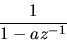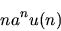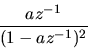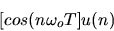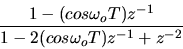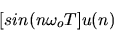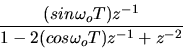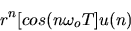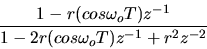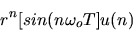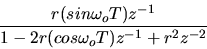## Z Transforms of Common Sequences

Ele 541 Electronic Testing

Unit Pulse

The z-transform of the sequence x(n) is defined to be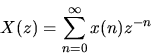If x(n) =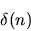, where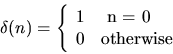then only the k = 0 term in the sum is non zero.
The z-transform of the unit pulse,= 1.

Unit Step

Consider the unit step function where x(k) =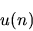Plugging into the definition of the z-transform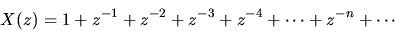This sum can be written as,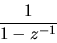Exponential Sequence

Consider the unit step function where x(k) =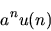Plugging into the definition of the z-transform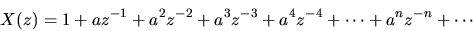This sum can be written as,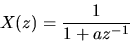Z-Transforms of Common Sequences

 Sequence z Transform1